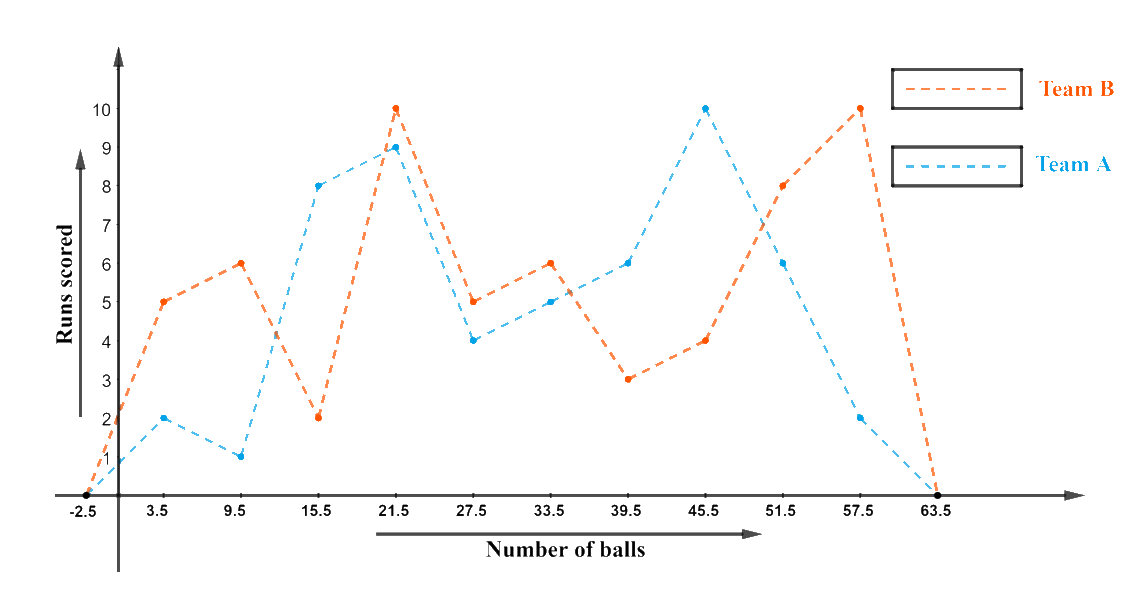# Ex.14.3 Q7 Statistics Solution - NCERT Maths Class 9

Go back to  'Ex.14.3'

## Question

The runs scored by two teams $$A$$ and $$B$$ on the first $$60$$ balls in a cricket match are given below:

 Number of balls Team A Team B $$1 – 6$$ $$2$$ $$5$$ $$7 – 12$$ $$1$$ $$6$$ $$13 – 18$$ $$8$$ $$2$$ $$19 – 24$$ $$9$$ $$10$$ $$25 – 30$$ $$4$$ $$5$$ $$31 – 36$$ $$5$$ $$6$$ $$37 – 42$$ $$6$$ $$3$$ $$43 – 48$$ $$10$$ $$4$$ $$49 – 54$$ $$6$$ $$8$$ $$55 – 60$$ $$2$$ $$10$$

Represent the data of both the teams on the same graph by frequency polygons.

[Hint: First make the class intervals continuous.]

Video Solution
Statistics
Ex exercise-14-3 | Question 7

## Text Solution

What is known?

The runs scored by two teams $$A$$ and $$B$$ on the first $$60$$ balls in a cricket match are given.

What is Unknown?

A frequency polygon to represent the data of both the teams.

Reasoning:

1. It can be observed from the given data that the class intervals of the given data are not continuous. There is a gap of ‘$$I$$’ unit between them. So, to make the class intervals continuous, $$0.5$$ has to be added to every upper class limit and $$0.5$$ has to be subtracted from the lower class limit.
2. Class mark should also be found as below:

\text{Class}\,\text{Marks}=\frac{\left( \begin{align} & \text{Upper}\,\text{Limit+} \\ & \text{Lower}\,\text{Limit} \\ \end{align} \right)}{2}

Steps:

The data table with continuous interval and with class mark is as below:

 Number of balls Class Mark Team A Team B $$0.5 \,– 6.5$$ $$3.5$$ $$2$$ $$5$$ $$6.5 \,– 12.5$$ $$9.5$$ $$1$$ $$6$$ $$12.5\, – 18.5$$ $$15.5$$ $$8$$ $$2$$ $$18.5\, – 24.5$$ $$21.5$$ $$9$$ $$10$$ $$24.5 \,– 30.5$$ $$27.5$$ $$4$$ $$5$$ $$30.5 \,– 36.5$$ $$33.5$$ $$5$$ $$6$$ $$36.5 \,– 42.5$$ $$39.5$$ $$6$$ $$3$$ $$42.5 \,– 48.5$$ $$45.5$$ $$10$$ $$4$$ $$48.5\,– 54.5$$ $$51.5$$ $$6$$ $$8$$ $$54.5 \,– 60.5$$ $$57.5$$ $$2$$ $$10$$

The frequency polygon for the above data can be constructed by

(i) Number of balls on $$x$$-axis

(ii) Runs scored on $$y$$-axis with an approximate scale of “$$1\,\rm unit = 1\,\rm run$$” as the lowest run was at $$1$$ and the highest was at $$10.$$Learn from the best math teachers and top your exams

• Live one on one classroom and doubt clearing
• Practice worksheets in and after class for conceptual clarity
• Personalized curriculum to keep up with school